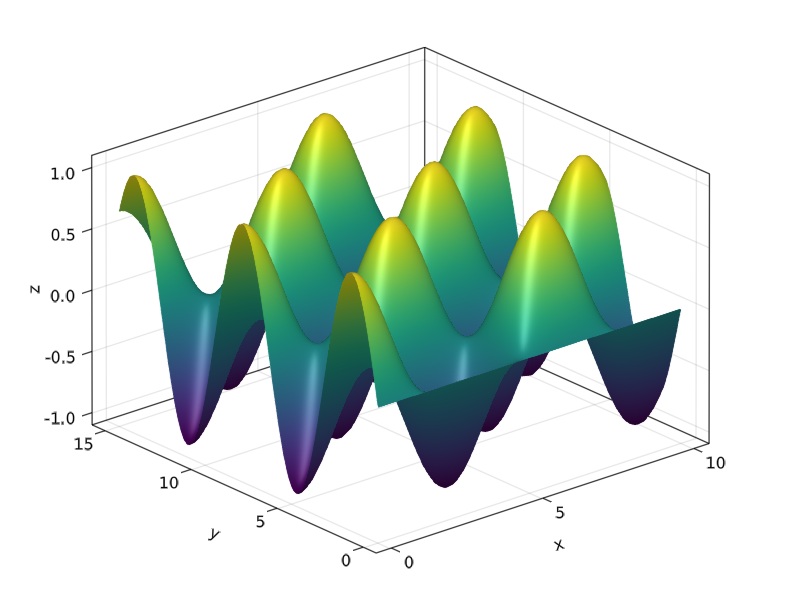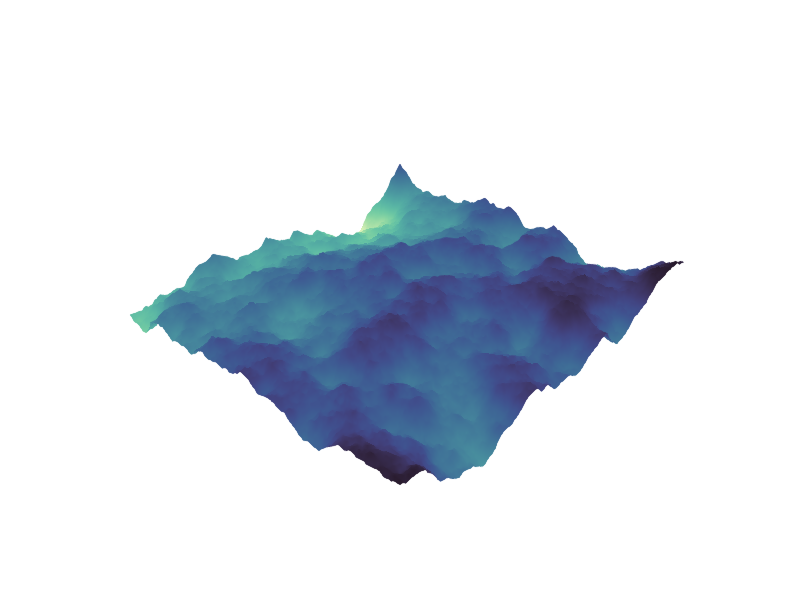# surface

MakieCore.surfaceFunction
surface(x, y, z)

Plots a surface, where (x, y) define a grid whose heights are the entries in z. x and y may be Vectors which define a regular grid, or Matrices which define an irregular grid.

source

### Examples

using GLMakie

xs = LinRange(0, 10, 100)
ys = LinRange(0, 15, 100)
zs = [cos(x) * sin(y) for x in xs, y in ys]

surface(xs, ys, zs, axis=(type=Axis3,))using SparseArrays
using LinearAlgebra
using GLMakie

# This example was provided by Moritz Schauer (@mschauer).

#=
Define the precision matrix (inverse covariance matrix)
for the Gaussian noise matrix.  It approximately coincides
with the Laplacian of the 2d grid or the graph representing
the neighborhood relation of pixels in the picture,
https://en.wikipedia.org/wiki/Laplacian_matrix
=#
function gridlaplacian(m, n)
S = sparse(0.0I, n*m, n*m)
linear = LinearIndices((1:m, 1:n))
for i in 1:m
for j in 1:n
for (i2, j2) in ((i + 1, j), (i, j + 1))
if i2 <= m && j2 <= n
S[linear[i, j], linear[i2, j2]] -= 1
S[linear[i2, j2], linear[i, j]] -= 1
S[linear[i, j], linear[i, j]] += 1
S[linear[i2, j2], linear[i2, j2]] += 1
end
end
end
end
return S
end

# d is used to denote the size of the data
d = 150

# Sample centered Gaussian noise with the right correlation by the method
# based on the Cholesky decomposition of the precision matrix
data = 0.1randn(d,d) + reshape(
cholesky(gridlaplacian(d,d) + 0.003I) \ randn(d*d),
d, d
)

surface(data; shading=false, colormap = :deep, axis = (show_axis = false,))